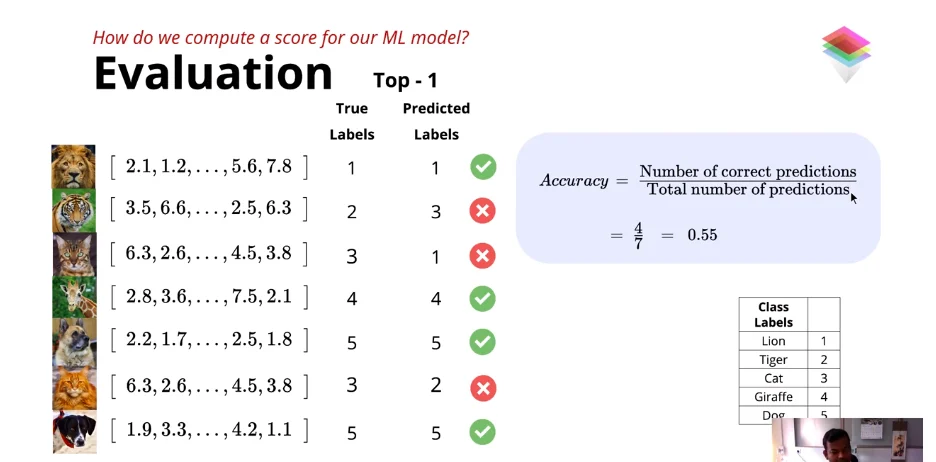# 6 Elements Of Machine Learning - A Beginner's Guide to Machine Learning

Note : Content of this article including some images are taken from the Deep Learning Course by One Fourth Labs.

Before introducing the 6 elements of Machine Learning, let's understand Why do we need Machine Learning? Why not the old school expert systems where we used to come up with a solution and then code it in one of our favourite programming languages for the computer to understand it and then feeding it some input to get the desired result? A simple example of such expert systems is "Whether a patient has dengue or not?" Now, how do doctors decide that? They ask the patient "Do they have high fever and cold? Do thay have headache or rashes?" i.e. Whether the patient has symptoms of Dengues or not? And from the past experience doctor decides "If the patient has Dengue or not?"

Now, How do we solve this problem using a computer? We can simply write some if-else conditions to get the output as shown below.

``````if HIGH_FEVER and COLD:
return no_dengue
elif HIGH_FEVER and VOMITING and not RASH:
return no_dengue
elif HIGH_FEVER and VOMITING and RASH:
return dengue``````

And now we can simply run this program and feed it with the symptoms of a new patient and can decide "Whether he/she has dengue or not?" Cool, right? So Why do we need Machine Learning?

The answer is the limitations of the expert systems that we talked about. Predicting dengue or not was somewhat easy task as we know about it's symptoms but let's say we want to predict whether a patient has Ebola or not? In this case, the symptoms(rules to check) are unknown and thus we can't feed the computer the rules that we don't know.

Another limitations is that sometimes we have lots of data. For example, "Should a bank give you a loan or not?" Now, giving loan depends on lots of factors including your salary, your credit score, size of your family and many more. Now in this case, we have so much data to make sense from that writing if-else blocks is not an easy and effective task.

And sometimes, there are rules that we can't express to the computer to understand. For example, sometimes your salary may be a little less than the required salary to get loan but you've been an honest and a regular customer to the bank and the bank manager knows that though your salary is not enough to meet the minimum requirements but they believe you and your honesty and gives you loan anyway.How do you feed such rule to the computer? How can a computer understand honesty?

These are the limitations that needs to be conquered and Machine Learning Does exactly that. Using Machine Learning, we give some data as input and some matching output for that data and then find the relationship between the input data and output data. The 6 elements of the the Machine Learning are: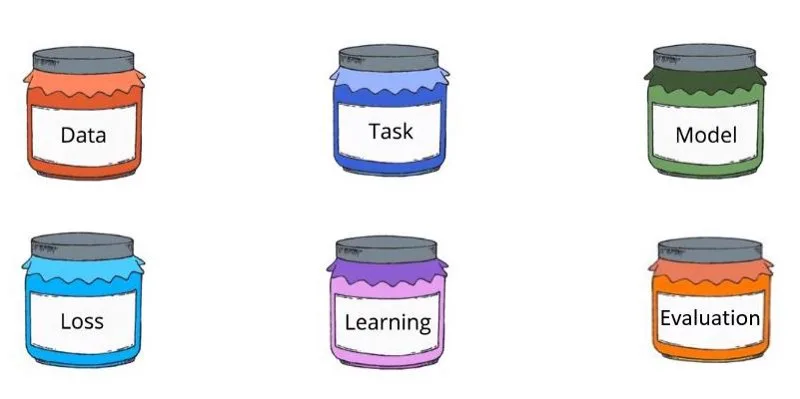Now, let's dive deep into these elements one by one and see how we can see Machine Learning through the lens of these elements.

## 1. Data

Data simply means information. All types and formats of information. Today,there is enormous amount of data produced every second and this data can be used to answer so many questions. There is text data as well as audio-video data, there is structured data as well as unstructured data.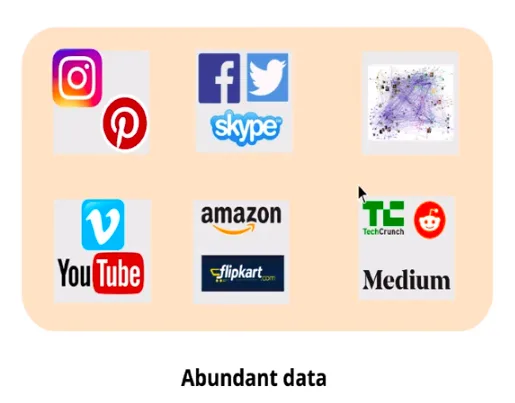Data is the fuel of Machine Learning.

When starting a Machine Learning project, we need some answers to the questions like, "Do we have the required data? If no, how do we get the data and where from? And if yes, is it in a structured form or unstructured and raw?"

These questions lead us to different paths in the project. If we don't have the data needed, then Data Curation comes in picture. There are many ways to get the data.

If you are lucky enough, there are many resources where you can get datasets that are publically available. Few examples of such resources are Google Dataset Search, Kaggle Datasets, Indian Government Data.

If you are rich enough, there are many websites on which you can assign some people to curate and collect data for your needs. For example, let's say you have images of animals and you want these images to be labelled with the name of animal, then you can assign such task to people and you can get the data easily and in a short span of time. Few examples of such crowdsourcing marketplaces are Amazon mturk, Dataturks.

And If you are smart enough, you can conduct surveys and get data from people or using the data scrapping techniques you can collect data from different websites.

One important thing to remember is that it doesn't matter in which format you get the data, at the end all the data needs to be encoded as numbers before feeding it to the computers. For example, images can be converted into matrix of pixel values.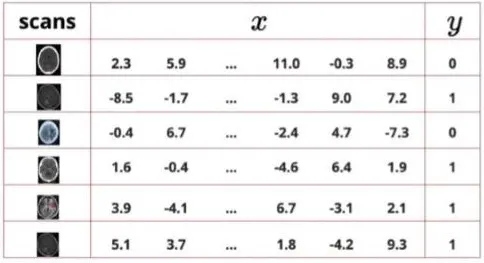Now, you have data. The next question you should ask is "What task do I need to achieve? Or What task can I achieve using the data that I have?"

Generally, Machine Learning tasks are divided into two parts: Supervised Learning And Unsupervised Learning. Supervised Learning is when you have some input data as well as some output data corresponding to the input data. Then you can use Machine Learning to get the relationship between the input and output data and then use that relationship to predict output for the new input. And Unsupervised Learning is when you have only input data.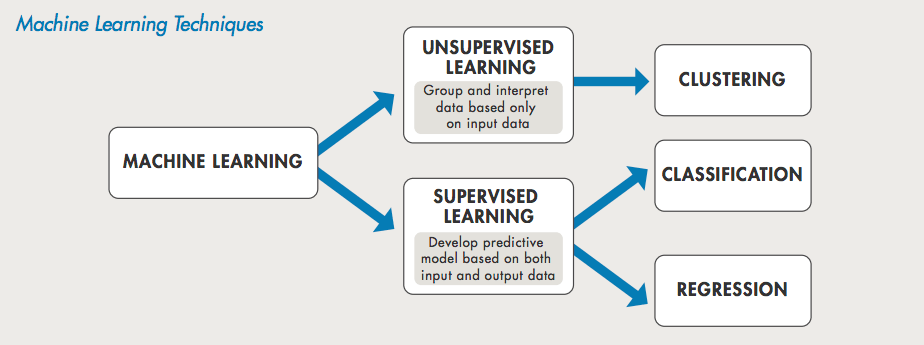Supervised learning is further divided into two sub sections as Regression and Classification where Regression means you want to predict some continous value for example House Price Prediction and Classification means you want a discrete answer such as yes or no. An example of Classification is, given an image you want to detect whether there is a dog or not in that image.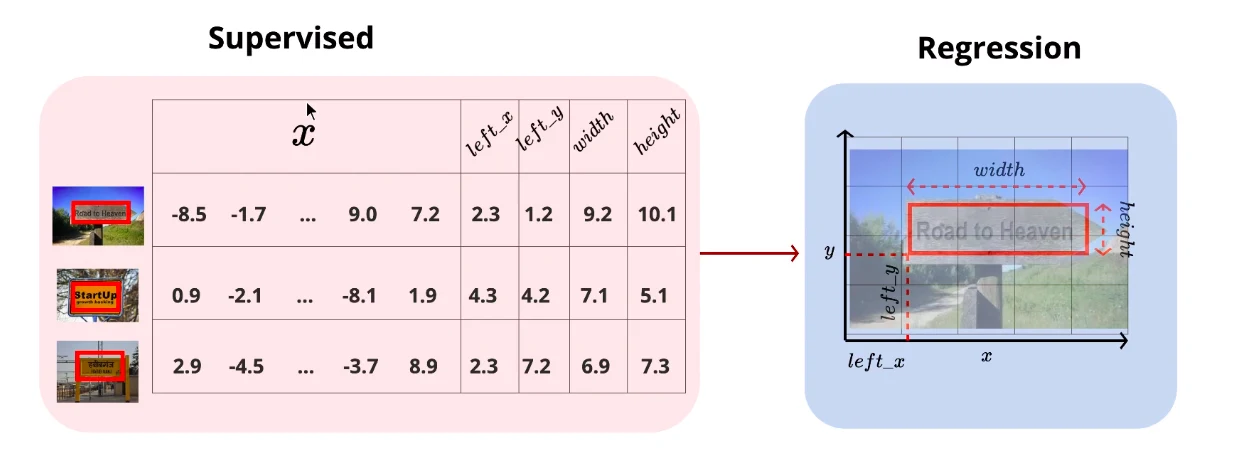Unsupervised learning is often used for Generation and Clustering. For example, the image given below shows that given the tweets of US President Donald Trump as an input, machine generates more such tweets.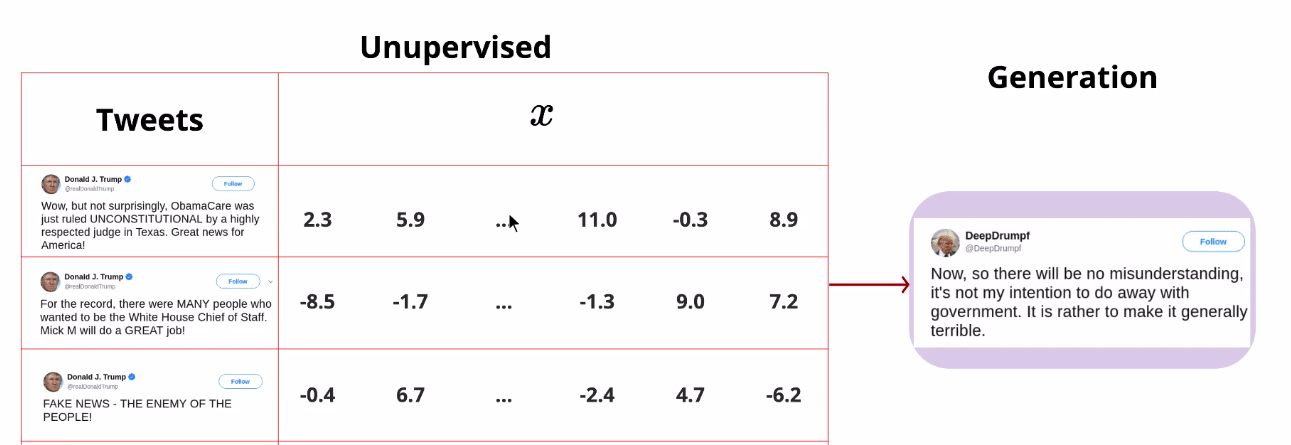Although Unsupervised learning is useful in some cases, most of the real world problems are solved using Supervised learning as it gives more accurate results than Unsupervised learning.

"Supervised learning has created 99% of economic value in AI." - Andrew NG, Co-founder of Coursera.

## 3. Model

A model is nothing but a mathematical function which defines relationship between input data and output data.

A model can be a simple Linear Function such as `y = mx + c` or it can be a complex function such as `y = sin(cos(ax)) + tan(sin(bx^2 + cx))` .

The example that we talked about before, given an image(data), predict if it has a dog or not(task), we have to build a system that answers this question and such system is called a model.

There are many models available that are created over the years and each one has it's own pros and cons and each one is suitable for a unique task. For a human, it is almost impossible to come up with a function that defines relationship between the input and output, given that the input data has over thousand different features.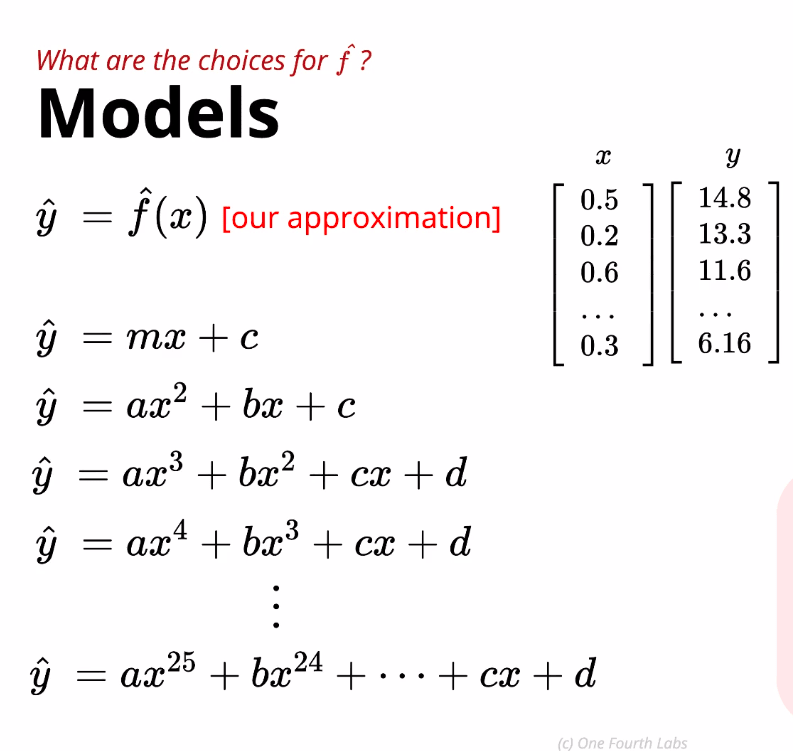## 4. Loss Function

Although, it's not possible to come up with a model just by looking at the given data, but let's assume, you have come up with a model that you think correctly defines the relationship between input and output. But, two of your friends are solving the same problem and they have come up with a slightly different model, then how do you decide, which model is better? Loss function gives the answer to that question.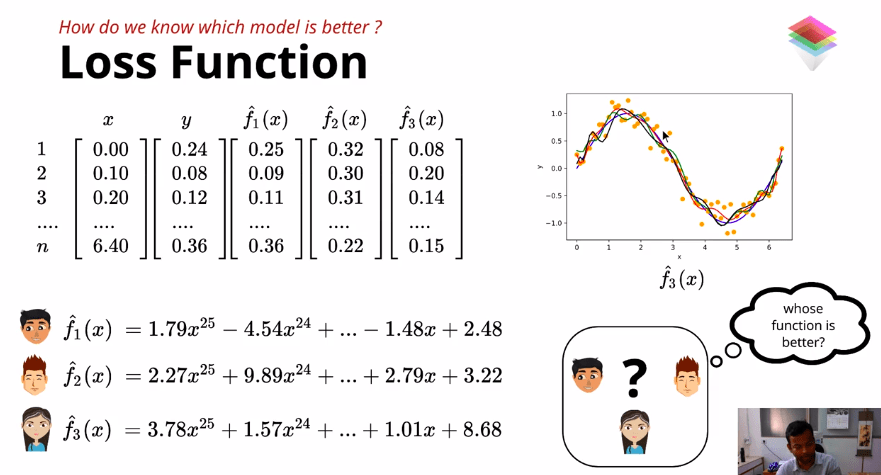As shown in the image above, three different values predicted by the models that you and your friends have written are `f1(x), f2(x) and f3(x)`, respectively. And the true value is `y`. Now, to know which model is best suited for our data, we compute the difference between the true value and predicted value for all the data using a loss function. One such loss function is Square Error Loss.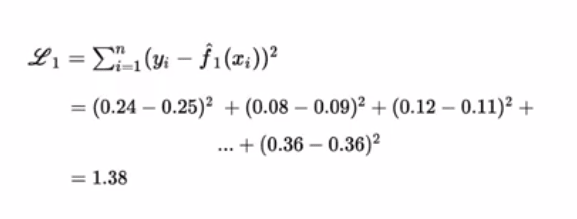And counting loss function value for all three models, we get to know that the best model for our data is the one with the minimum value of loss function which is `1.38`.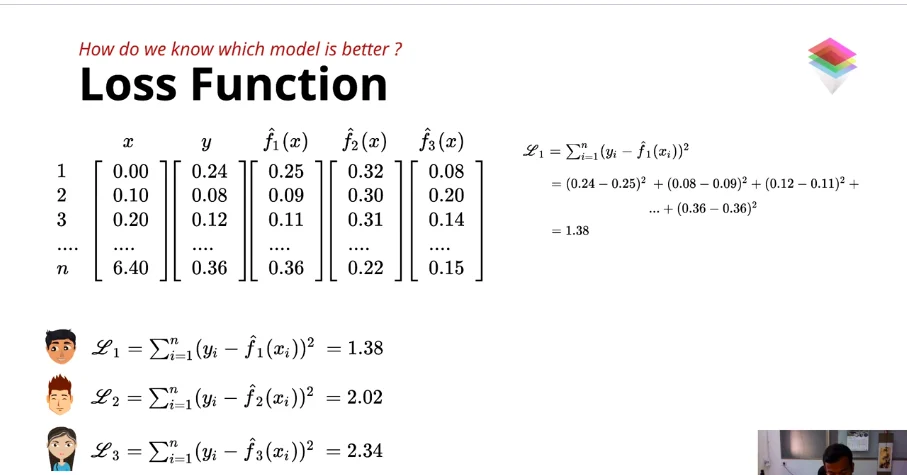This way using a loss function, we can get the best model that defines the relationship between our input data and output data. More such examples of Loss Function are Cross Entropy Loss and KL Divergence.

## 5. Learning Algorithm

Now, we assumed a hypothetical situation where you and your friends came up with a model for our data and task but that won't happen in reality as there will be hundred and thousands of features in the data and you can't just look at it and propose a model that satisfies the relationship. Instead, you can propose a function such as `y = ax^3 + bx^2 + cx` and say that I think this should define the relationship between the input x and output y, but you don't know the paramteres a, b and c. So, to identify these parameters for a model, we need a learning algorithm.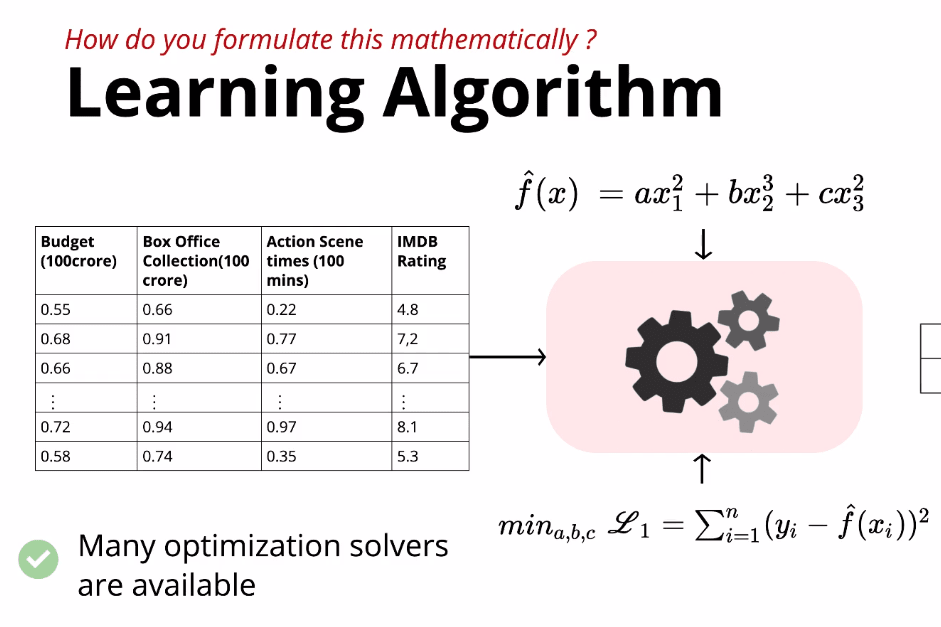As shown in the picture above, we have a model with three parameters a,b and c and we need values of a,b and c at which the loss function's value is minimum. In calculus, there are many optimization strategies available that we can use to find the best values of parameters which minimizes the loss. Some examples of such optimization solvers are Gradient Descent, Adagrad, RMSProp, etc.

Learning algorithm is one task that computer does, other than that from data collection to deciding task and then proposing a suitable model is done by humans but then finding parameters of the proposed model is done by computer.

## 6. Evaluation

Okay,let's say using the above 5 elements, you've created an ML model to detect the animal in the image. And you want to sell it to some company or just show off to your friends. How do you know that your model is detecting the right animal? You guessed it right! you have to test your model. That's what evaluation is. Testing your model by feeding it some test data and then checking if it predicts the correct output in all the cases? If not, then how accurate your model is?

Note: You evaluate your model using test data and not the training data, but why so? that's beyond the scope of this article!

There are many performance matrices available for evaluation, one such matrix is accuracy. We find the accuracy of a model `diving "Number of correct predictions" by "Total numbers of predictions"` , as shown in the image below.